Perry Sprawls, Ph.D.Online Textbook Table of Contents

 CHAPTER CONTENTS
 INTRODUCTION AND OVERVIEW ENERGY FORMS AND CONVERSION RADIATION Particle Radiation ENERGY UNITS AND RELATED QUANTITIES Power Intensity Potential Energy Energy Exchange ELECTRICAL QUANTITIES Current Power Total Energy

# INTRODUCTION AND OVERVIEW

There are two components of the physical universe: energy and matter. In most physical processes there is a constant interaction and exchange between the two; medical imaging is no exception. In all imaging methods, images are formed by the interaction of energy and human tissue (matter). A variety of energy types are used in medical imaging. This is, in part, what accounts for the difference in imaging methods. In this chapter we review some basic energy concepts and then look in detail at radiation, which is energy on the move, and the role of electrons in energy transfer.

Images of internal body structures require a transfer of energy from an energy source to the human body and then from the body to an appropriate receptor, as shown here.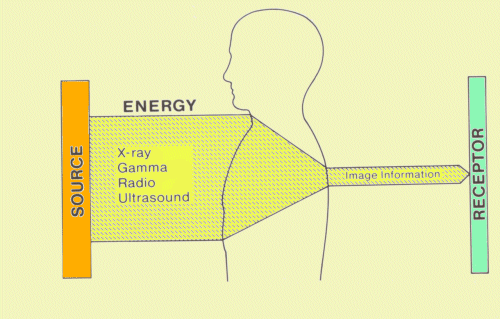Role of Energy in Medical Imaging

Although the types might be different, certain characteristics apply to all energy used in imaging.

A basic requirement is that the energy must be able to penetrate the human body. Visible light is the primary type of energy used to transfer image information in everyday life.  However, because it usually cannot penetrate the human body, we must use other energy types for internal body imaging.

Another characteristic of any energy used for imaging is that it must interact with internal body structures in a manner that will create image information.

A common element of all imaging methods is that a large portion of the energy used is deposited in the human tissue.  It does not reside in the body as the same type of energy but is converted into other energy forms such as heat and chemical change.  The possibility that the deposited energy will produce an undesirable biological effect must always be considered.

As we approach the process of medical imaging, it is helpful to recognize two broad categories of energy.  One category is the group of energy forms that require a material in which to exist. The other category is energy that requires no material object for its existence. Although the latter category does not require matter for its existence, it is always created within a material substance and is constantly moving and transferring energy from one location to another. This form of energy is radiation; all energy forms used for medical imaging, with the exception of ultrasound, are forms of radiation.

# ENERGY FORMS AND CONVERSION

The significance of matter-related energy forms in medical imaging is that they supply the energy to form radiation and later recapture it when the radiation is absorbed.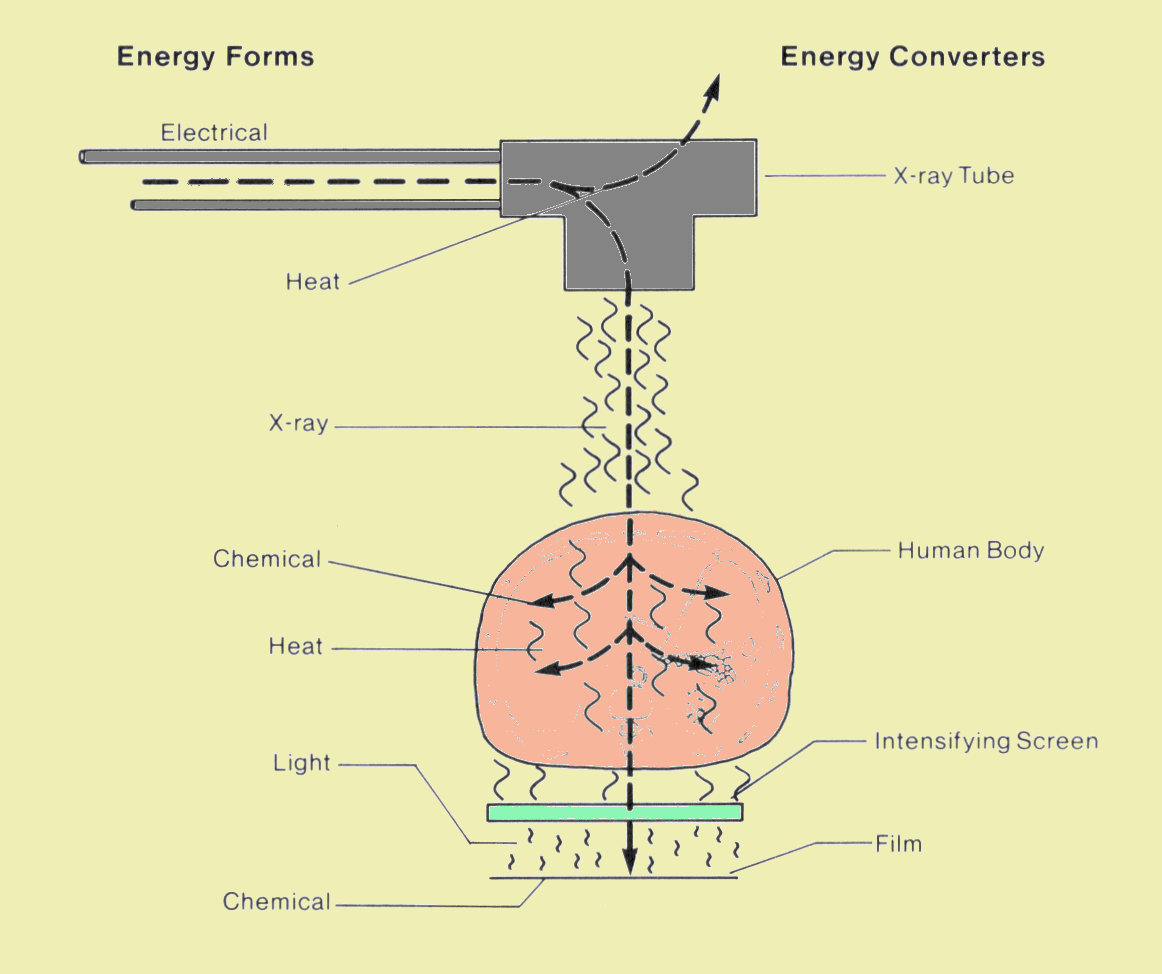Forms of Energy Involved in the Production of an X-Ray Image

A basic physical principle of the universe is that energy can be neither created nor destroyed.  However, we can transform it from one form or type to another.  Here we see some of the energy forms used in the production of an x-ray image.  Various components of the imaging system convert the energy from one form to another.

Radiation is energy that moves through space from one object, the source, to another object where it is absorbed. Radiation sources are generally collections of matter or devices that convert other forms of energy into radiation.  In some cases the energy to be converted is stored within the object.  Examples are the sun and radioactive materials.  In other cases the radiation source is only an energy converter, and other forms of energy must be applied in order to produce radiation; light bulbs and x-ray tubes are examples.

Most forms of radiation can penetrate through a certain amount of matter.  But in most situations, radiation energy is eventually absorbed by the material and converted into another energy form.

There are two general types of radiation, as shown here.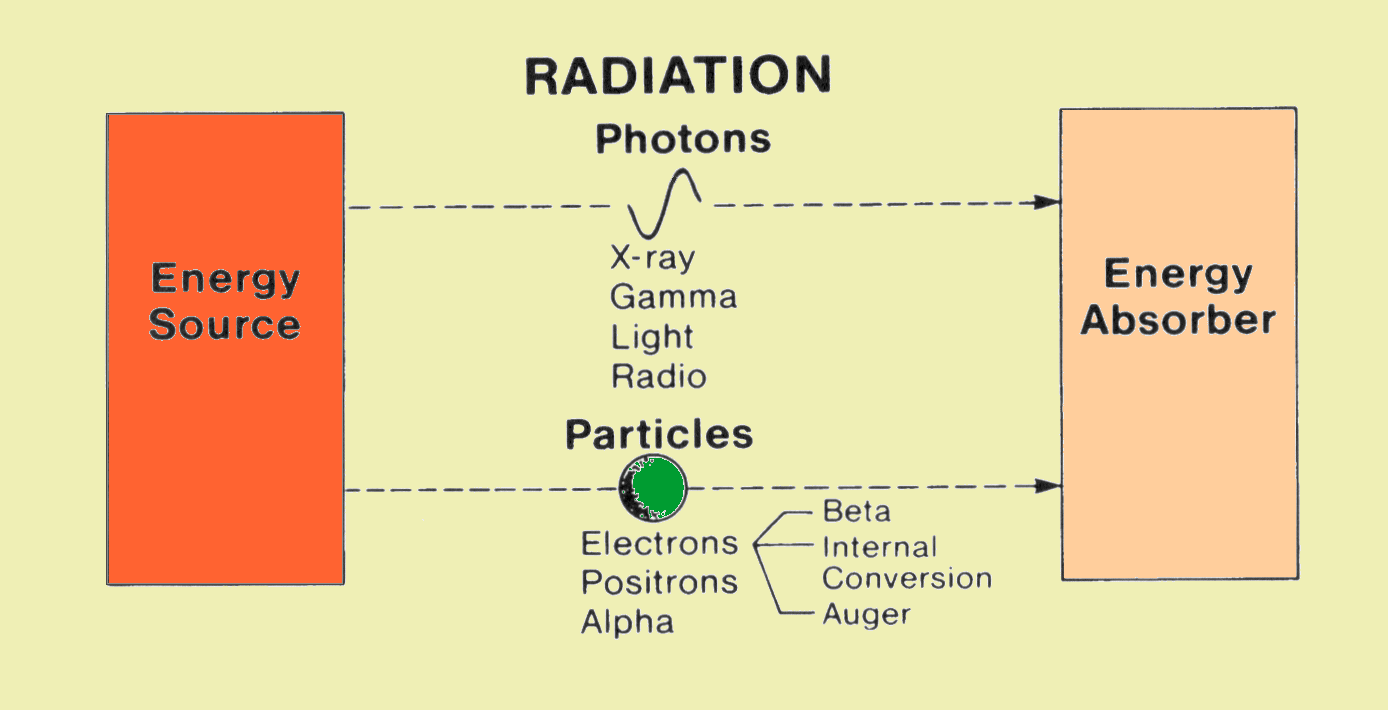Comparison of the Two Basic Types of Radiation

In one type, the energy is "packaged" in small units known as photons or quanta.  A photon or quantum of energy contains no matter, only energy.  Since it contains no matter, it has no mass or weight. This type of radiation is designated electromagnetic radiation.  Within the electromagnetic radiation family are a number of specific radiation types that are used for different purposes.  These include such familiar radiations as radio signals, light, x-radiation, and gamma radiation. The designations are determined by the amount of energy packaged in each photon.

The other general type of radiation consists of small particles of matter moving through space at a very high velocity. They carry energy because of their motion.  Particle radiation comes primarily from radioactive materials, outer space, or machines that accelerate particles to very high velocities, such as linear accelerators, betatrons, and cyclotrons.  Particle radiation differs from electromagnetic radiation in that the particles consist of matter and have mass. The type of particle radiation encountered most frequently in clinical medicine is high-velocity electron radiation.  Particle radiation is generally not used as an imaging radiation because of its low tissue penetration.  Also, when x-radiation interacts with matter, such as human tissue, it transfers energy to electrons, thus creating a form of electron radiation within the material.  Several types of particle radiation are produced as byproducts of photon production by a number of radioactive materials used in medical imaging.

# ENERGY UNITS AND RELATED QUANTITIES

There are occasions on which we must consider the quantity of energy involved in a process.  Many units are used to quantify energy because of the different unit systems (metric, British, etc.) and the considerable range of unit sizes. At this time, we consider only those energy units encountered in radiological and medical imaging procedures. The primary difference among the energy units to be considered is their size, which in turn determines their specific usage. We use the basic x-ray system as shown here to introduce the various energy units.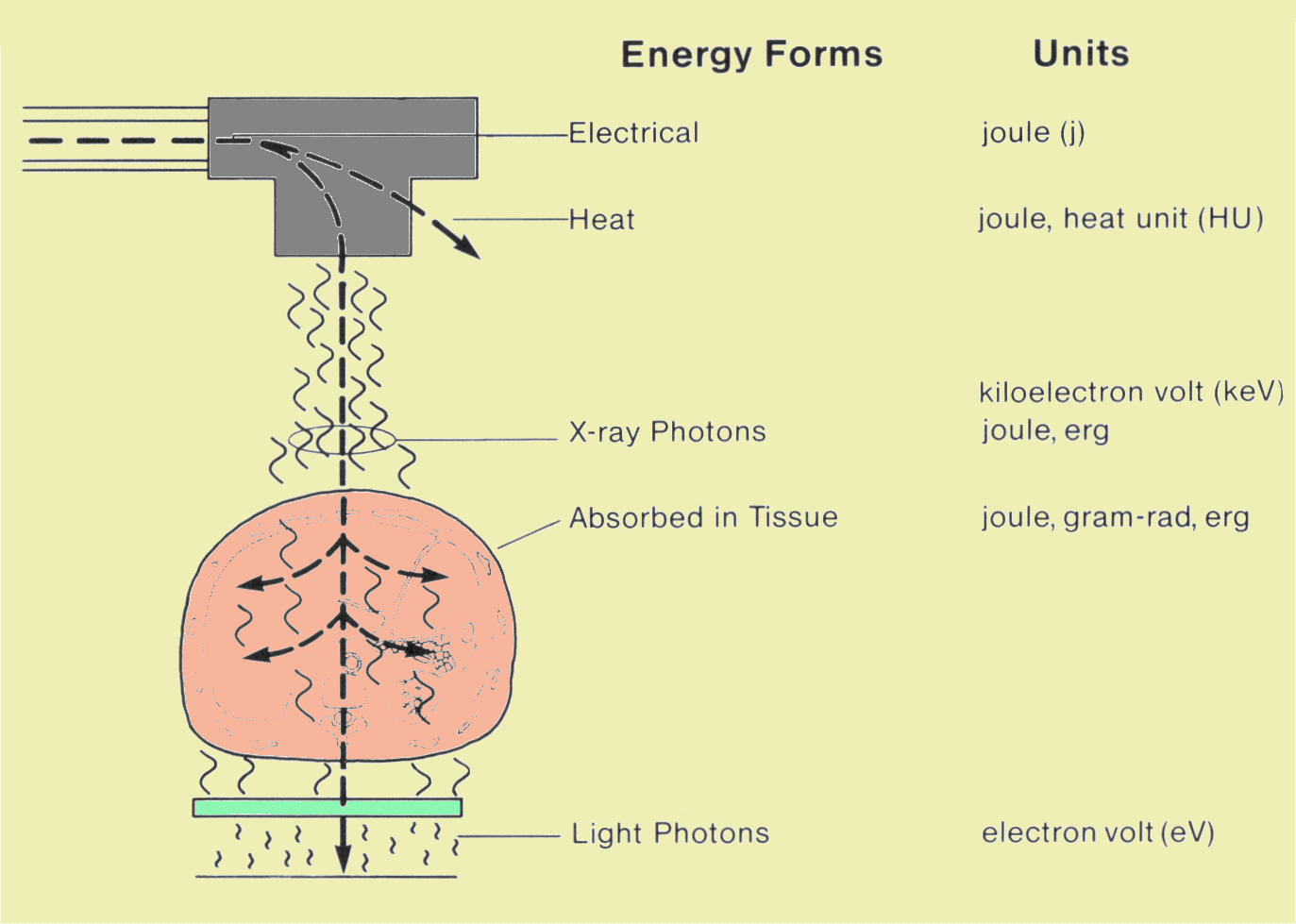Energy Units Encountered in X-Ray Imaging

Joule

The joule (J) is the fundamental unit of energy in the metric International System of Units (SI*). It is the largest unit of energy encountered in radiology. One joule is equivalent to 1 watt second. A 100-watt light bulb dissipates 100 J of energy per second.  In the next chapter we consider the full range of quantities and units used specifically for radiation; several are energy-related and are defined in terms of the joule or other energy units.

In general the joule is used when relatively large quantities of energy are involved.

*From the French name, Le Systeme International d'Unites.

# Heat Unit

The heat unit was developed within radiology as a convenient unit for expressing the amount of heat energy produced by an x-ray tube.  One heat unit is 71% of a joule.  The use of the heat unit is discussed in the Chapter on X-ray Tube Heating and Cooling; it is gradually being replaced by the joule.

The gram-rad is another unit developed in radiology to express the total radiation energy absorbed by the body. Its usage is discussed in the following chapter.  A general trend is to use the joule for this application rather than the gram-rad.

# Erg

The erg is a metric energy unit but is not an SI unit.  It is much smaller than the joule.  Its primary use in radiology is to express the amount of radiation energy absorbed in tissue.

# Electron Volt

The electron volt (eV) is the smallest energy unit.  It and its multiples, kiloelectron volt (keV) and megaelectron volt (MeV), are used to express the energy of individual electrons and photons.  The energy of individual light photons is in the range of a few electron volts.  X-ray and gamma photons used in imaging procedures have energies ranging from approximately fifteen to several hundred kiloelectron volts.

The relationships of the three basic energy units are

1 joule = 107 ergs

1 joule = 6.24 x 1018 electron volts.

# Power

Power is the term that expresses the rate at which energy is transferred in a particular process.  The watt is the unit for expressing power.  One watt is equivalent to an energy transfer or conversion at the rate of 1 J/sec.  As mentioned above, a 100-watt light bulb converts energy at the rate of 100 J/sec.  In medical imaging, power is used to describe:

•  the capability of x-ray generators,

•  the limitations of x-ray tubes,

•  the output of ultrasound transducers,

•  the rate at which energy is deposited in tissue during MRI procedures.

# Intensity

Intensity is the spatial concentration of power and expresses the rate at which energy passes through a unit area.  It is typically expressed in watts per square meter or watts per square centimeter.  Intensity is also used to express relative values of x-ray exposure rate, light brightness, radio frequency (RF) signal strength in MRI, etc.

# THE QUANTUM NATURE OF RADIATION

We have seen that electromagnetic radiation is packaged as individual photons or quanta.  This is sometimes referred to as the quantum nature of radiation and becomes an important concept in understanding how radiation is created and absorbed.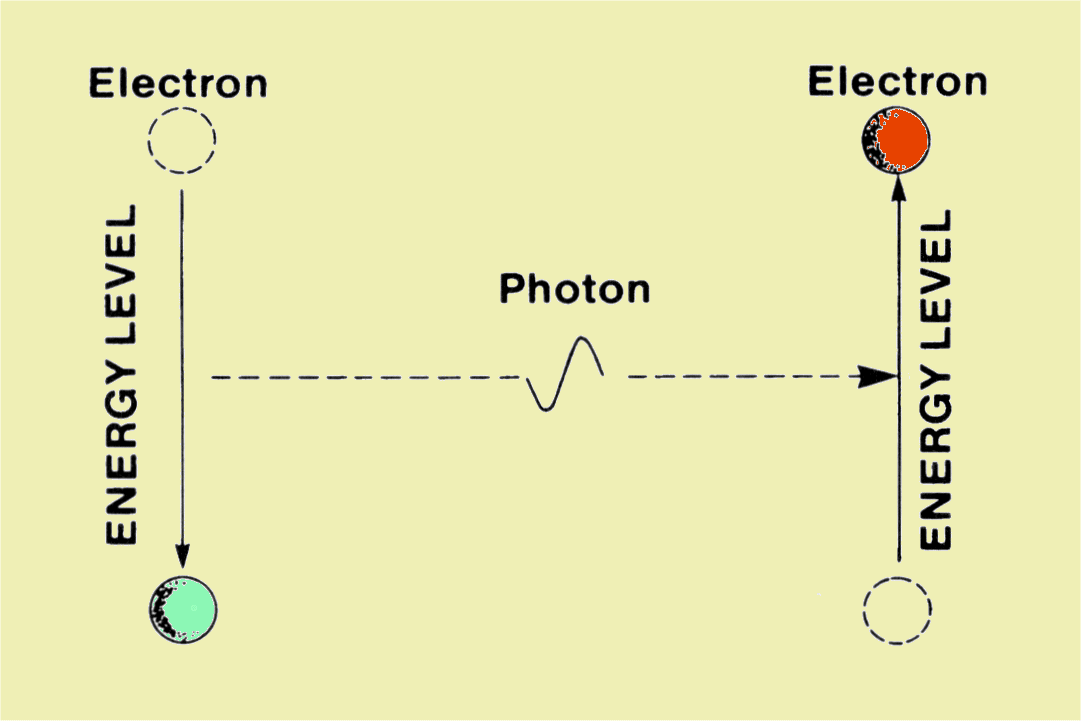The Quantum Nature of Radiation and Matter

Here we see an illustration of the basic quantum characteristics of both radiation and matter.  When we consider the structure of matter in another chapter we will find that electrons within atoms generally reside at specific energy levels rather than at arbitrary energy levels.  Electrons can move from one energy level to another, but they must go all the way or not at all.  These discrete electron energy levels give matter certain quantum characteristics.  In simple terms, matter prefers to exchange energy in predefined quantities rather than in arbitrary amounts.  Radiation travels through space as a shower of individual photons.

Eventually the photon is absorbed by transferring its energy back to an electron.  The chance of its absorption is greatly enhanced if it encounters a material with electron energy levels close to its energy content.  The important point here is that radiation photons are created and absorbed individually through energy exchanges within certain materials.

Although radiation photons are differentiated by several physical quantities, as shown here, all electromagnetic radiation travels with the same velocity through space.  Because light is one of the most common forms of electromagnetic radiation and its velocity is known, it is often said that electromagnetic radiations travel with the speed of light. In free space, this is a velocity of about 3 x 108 m/ sec.  If we assume that the average x-ray photon travels 1 m between the time it is created and the time it is absorbed, the average lifetime of a photon would be 3.3 x 10-9 seconds. Photons cannot be stored or suspended in space.  Once a photon is created and emitted by a source, it travels at this very high velocity until it interacts with and is absorbed by some material.  In its very short lifetime, the photon moves a small amount of energy from the source to the absorbing material.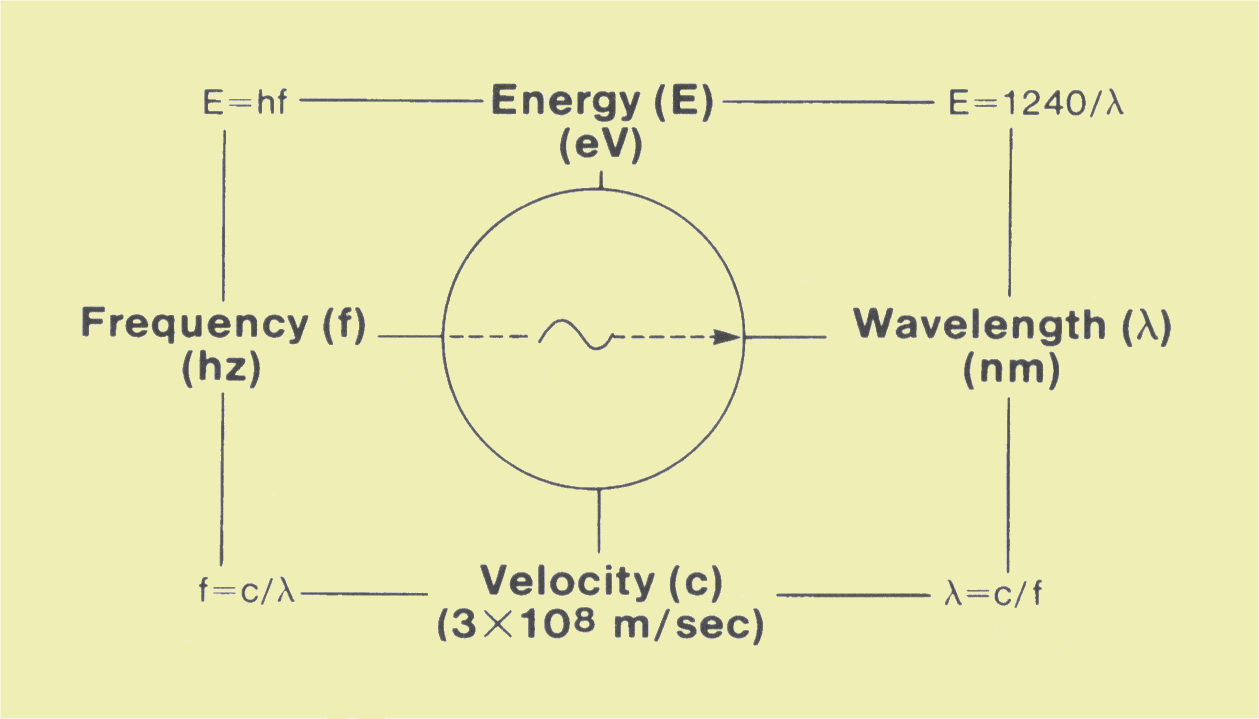Physical Characteristics of a Photon

Here we see the scales for the three quantities that are shown in relationship to the various types of radiation.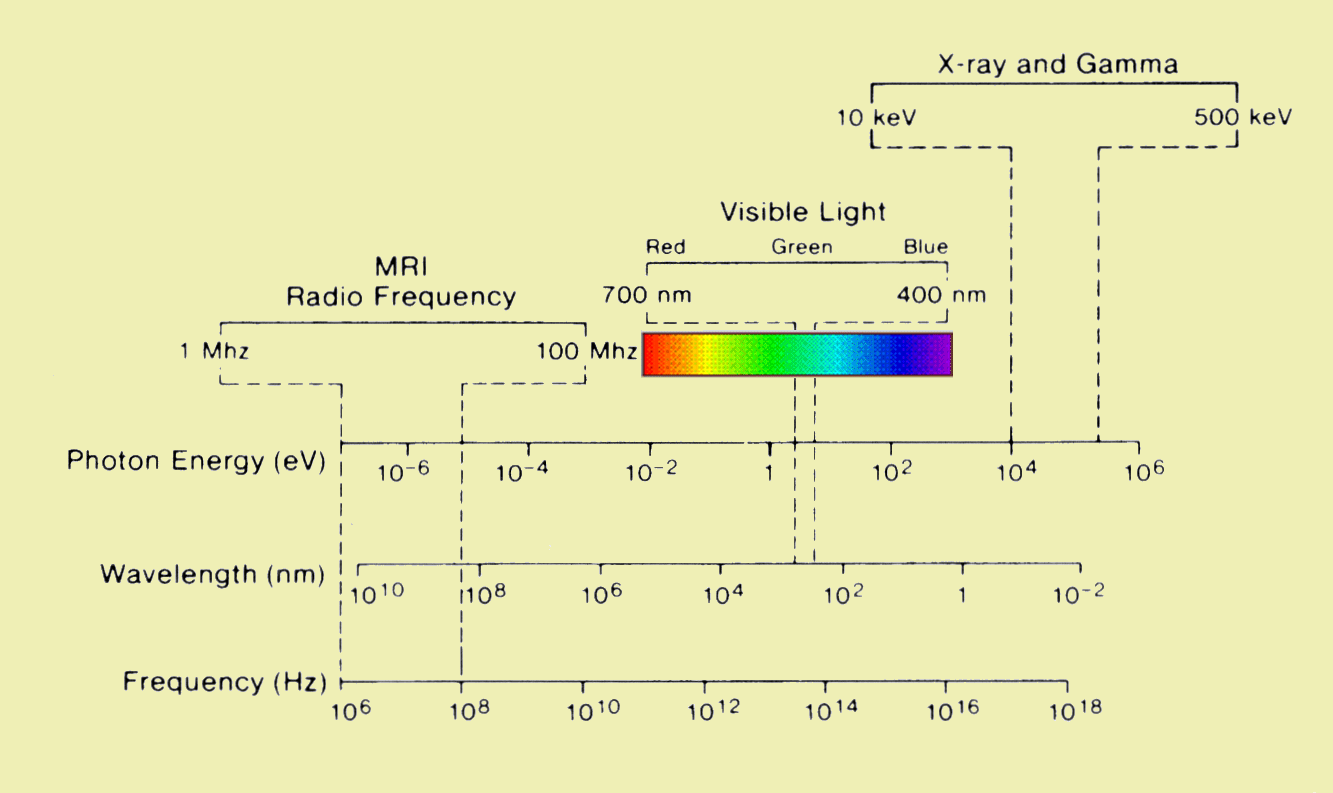The Electromagnetic Spectrum

While it is possible to characterize any radiation by its photon energy, wavelength, or frequency, the common practice is to use different quantities for different types of radiations, as discussed below.

Since a photon is simply a unit of energy, its most important characteristic is the quantity of energy it contains.  Photon energies are usually specified in units of electron volts or appropriate multiples.

If the various types of electromagnetic radiation were ordered with respect to photon energies, as shown above, the scale would show the electromagnetic spectrum.  It is the energy of the individual photons that determines the type of electromagnetic radiation: light, x-ray, radio signals, etc.

An important aspect of photon energy is that it generally determines the penetrating ability of the radiation.  The lower energy x-ray photons are often referred to as soft radiation, whereas those at the higher-energy end of the spectrum would be so-called hard radiation.  In most situations, high-energy (hard) x-radiation is more penetrating than the softer portion of the spectrum.

If the individual units of energy, photons or particles, have energies that exceed the binding energy of electrons in the matter through which the radiation is passing, the radiation can interact, dislodge the electrons, and ionize the matter.

# Frequency

Frequency is a rate of vibration or oscillation. One of the laws of physics that applies to electromagnetic radiation is that a photon's energy (E) and frequency (f) are directly proportional, with the relationship being

E = hf.

In this relationship, h is Planck's constant, which has a value of 6.625 x 10-27 erg-second, and f is frequency in hertz (Hz, cycles per second).

Frequency is the most common quantity used to characterize radiations in the lower end, or the RF portion, of the electromagnetic spectrum and includes radiation used for radio and television broadcasts, microwave communications and cooking, and MRI. For example, in MRI, protons emit signals with a frequency of 42.58 MHz when placed in a 1-tesla magnetic field. Although, theoretically, x-radiation has an associated frequency, the concept is never used.

The minimum radiation energy that can produce ionization varies from one material to another, depending on the specific electron binding energies. Electron binding energy is discussed in more detail in Chapter 4. The ionization energies for many of the elements found in tissue range between 5 eV and 20 eV. Therefore, all radiations with energies exceeding these values are ionizing radiations.

Photon energy quantities are generally used to describe radiation with relatively high photon energy, such as x-ray, gamma, and cosmic radiation.

Various physical phenomena observed with electromagnetic radiation suggest that the radiation has certain wavelike properties.  A characteristic of a wave is the distance between two successive peaks, which is the wavelength (λ).  This is also the distance the radiation moves forward during the period of one oscillation.  Wavelength can be expressed in any unit of length.  Radio and television signals have relatively long wavelengths that are usually expressed in meters.  For higher energy photons, such as light and x-ray, two smaller length units are used. These are:

1 Angstrom unit (Å) = 10-10m

1 nanometer (nm) = 10-9m

The relationship between photon energy and wavelength is

E (keV) = 1.24 / λ (nm)

This relationship allows the conversion of photon energy into wavelength and vice versa.  In some literature, x-ray photon spectra are given in terms of wavelength rather than photon energy.  This causes the spectrum curve to have an entirely different appearance.  By using the relationship given above, it is possible to convert a spectrum of one kind into the other.  Since energy and wavelength are inversely related, the highest energy on the spectrum corresponds to the shortest wavelength.

Wavelength is most frequently used to describe light.  At one time it was used to describe x-radiation but that practice is now uncommon.  Wavelength is often used to describe radio-type radiations.  General terms like "shortwave" and "microwave" refer to the wavelength characteristics of the radiation.

# ELECTRONS AND ENERGY

Electrons are the smallest particles found in matter.  An electron has a mass of 9.1 x 10-28 g, which means it would take 10.9 x 1026 electrons to equal the weight of 1 cm3 of water.  The question might be raised as to why such a small particle can be the foundation of our modern technology.  The answer is simple — numbers.  Tremendous numbers of electrons are involved in most applications.  For example, when a 100-watt light bulb is turned on, electrons race through the wires carrying energy to it at the rate of 5.2 x 1018 electrons per second.  In addition to its mass, each electron carries a 1-unit negative electrical charge.  It is the charge of an electron that enables it to interact with other electrons and particles within atoms.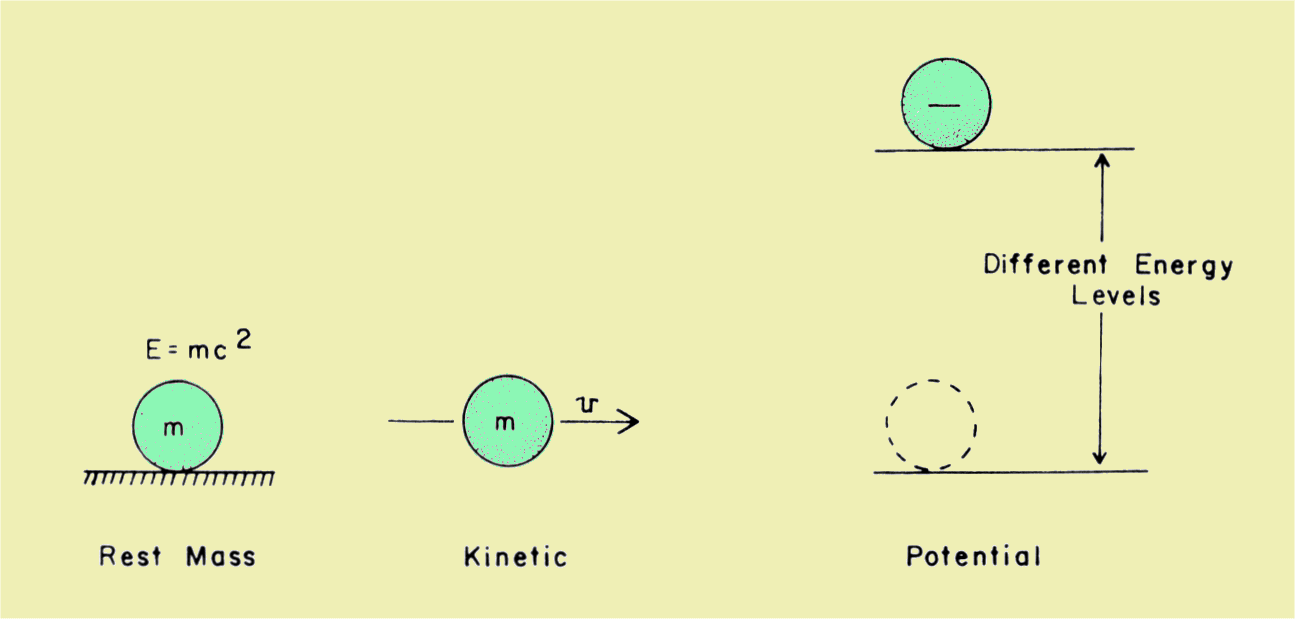Types of Energy Associated with Electrons

Because an electron has both mass and electrical charge, it can possess energy of several types, as shown here.  It is the ability of an electron to take up, transport, and give up energy that makes it useful in the x-ray system.

# Rest Mass Energy

Even when an electron is at rest and has no apparent motion, it still has energy.  In fact, according to the laws of physics, an object has some energy just because of its mass.  Under certain conditions, mass can be converted into energy and vice versa. Einstein's famous equation

E=mc2

predicts the amount of energy that could be obtained if an object with a mass, m, were completely converted.  In this relationship, c is the speed of light.  Although it is not possible with our present technology to convert most objects into energy, certain radioactive materials emit particles, called positrons, that can annihilate electrons.  When this happens, the electron's entire mass is converted into energy. According to Einstein's relationship, each electron will yield 510 keV.  This energy appears as a photon.  The annihilation of positrons and electrons is the basis for positron emission tomography (PET).

# Kinetic Energy

Kinetic energy is associated with motion.  It is the type of energy that a moving automobile or baseball has.  When electrons are moving, they also have kinetic energy.

Generally, the quantity of kinetic energy an object has is related to its mass and velocity.  For large objects, like baseballs and cars, the energy is proportional to the mass of the object and the square of the velocity.  Doubling the velocity of such an object increases its kinetic energy by a factor of 4.  In many situations, electrons travel with extremely high velocities that approach the velocity of light.  At these high velocities, the simple relationship between energy and velocity given above does not hold.  One of the theories of relativity states that the mass of an object, such as an electron, changes at high velocities.  Therefore, the relationship between energy and velocity becomes complex.  Electrons within the typical x-ray tube can have energies in excess of 100 keV and can travel with velocities of more than one-half the speed of light.

# Potential Energy

Potential energy is the type of energy possessed by an object because of its location or configuration and is essentially a relative quantity.  That is, an object will have more or less energy in one location or configuration than in another. Although there is generally not a position of absolute zero potential energy, certain locations are often designated as the zero-energy level for reference.

Electrons can have two forms of potential energy.  One form is related to location within an electrical circuit, and the other is related to location within an atom.  One important aspect of electron potential energy is that energy from some source is required to raise an electron to a higher energy level, and that an electron gives up energy when it moves to a lower potential energy position.

# Because electrons are too small to see, it is sometimes difficult to visualize what is meant by the various types of electron energy.  Consider the stone shown here; we will use it to demonstrate the various types of energy that also apply to electrons.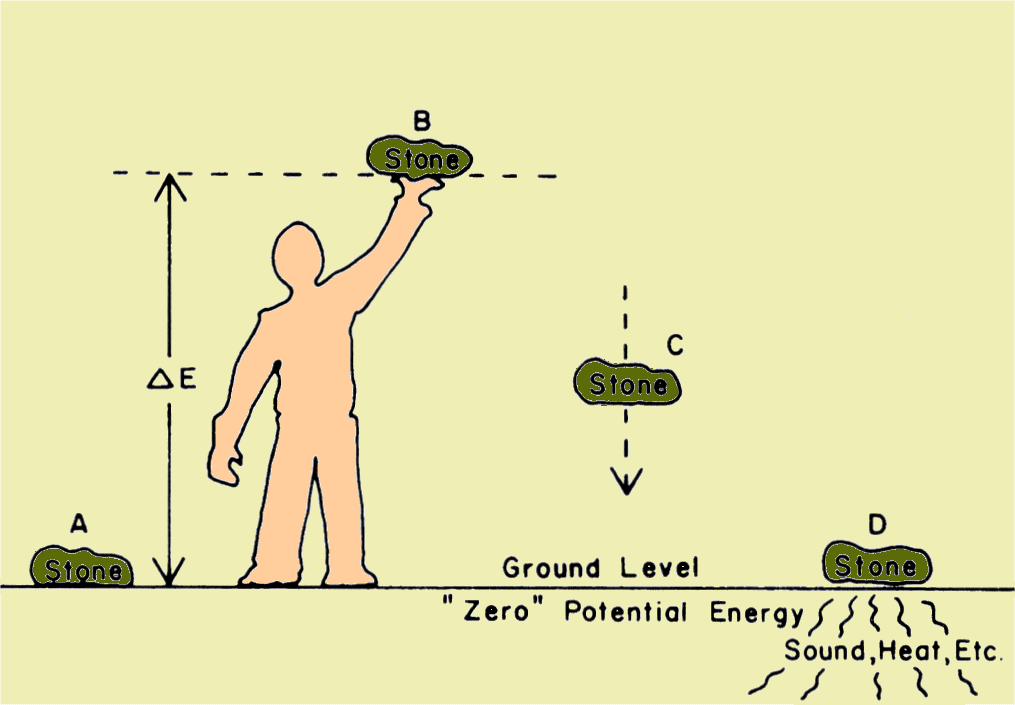Transfer of Energy from One Form to Another

Potential energy is generally a relative quantity.  In this picture, the ground level is arbitrarily designated as the zero potential energy position.  When the stone is raised above the ground, it is at a higher energy level.  If the stone is placed in a hole below the surface, its potential energy is negative with respect to the ground level.  However, its energy is still positive with respect to a position in the bottom of a deeper hole.  The stone at position A has zero potential energy (relatively speaking), zero energy because it is not moving, and a rest-mass energy proportional to its mass.  (The rest-mass energy of a stone is of no practical use and is not discussed further.)  When the man picks up the stone and raises it to position B, he increases its potential energy with respect to position A.  The energy gained by the stone comes from the man.  (We show later that electrons can be raised to higher potential energy levels by devices called power supplies.)  The additional potential energy possessed by the stone at B can be used for work or can be converted into other forms of energy.  If the stone were connected to a simple pulley arrangement and allowed to fall back to the ground, it could perform work by raising an object fastened to the other end of the rope.

If the man releases the stone at B and allows it to fall back to the ground, its energy is converted into kinetic energy.  As the stone moves downward, decreasing its potential energy, which is proportional to its distance above the ground, it constantly increases its speed and kinetic energy.  Just before it hits the ground, its newly gained kinetic energy will be just equal to the potential energy supplied by the man.  (Electrons undergo a similar process within x-ray tubes where they swap potential for kinetic energy.)  Just as the stone reaches the surface of the ground, it will have more energy than when it was resting at position A.  However, when it comes to rest on the ground at D, its energy level is the same as at A.  The extra energy originally supplied by the man must be accounted for.  In this situation, this energy is converted into other forms, such as sound, a small amount of heat, and mechanical energy used to alter the shape of the ground. When high-speed electrons collide with certain materials, they also lose their kinetic energy; their energy is converted into heat and x-radiation.

# Energy Transfer

One of the major functions of electrons is to transport energy from one location to another.  We have just seen that individual electrons can possess several forms of energy.  The principle of electrical energy transportation is that electrons pick up energy in one location and then move to another where they pass the energy on to some other material.  Generally the arrangement is such that the electrons then move back to the energy source and repeat the process.

The pathway electrons travel as they transfer energy from one point to another is a circuit.  A basic electrical circuit is shown here. All circuits must contain at least two components (or devices) as shown.  One component, designated here as the source, can convert energy from some other form and transfer it to the electrons. Batteries are good examples of electron energy sources.  The other component, designated here as a load, performs essentially the opposite function. As the electrons pass through the device they lose their energy as it is converted into some other form; a light bulb is a good example of a load in which its energy is converted into light and heat.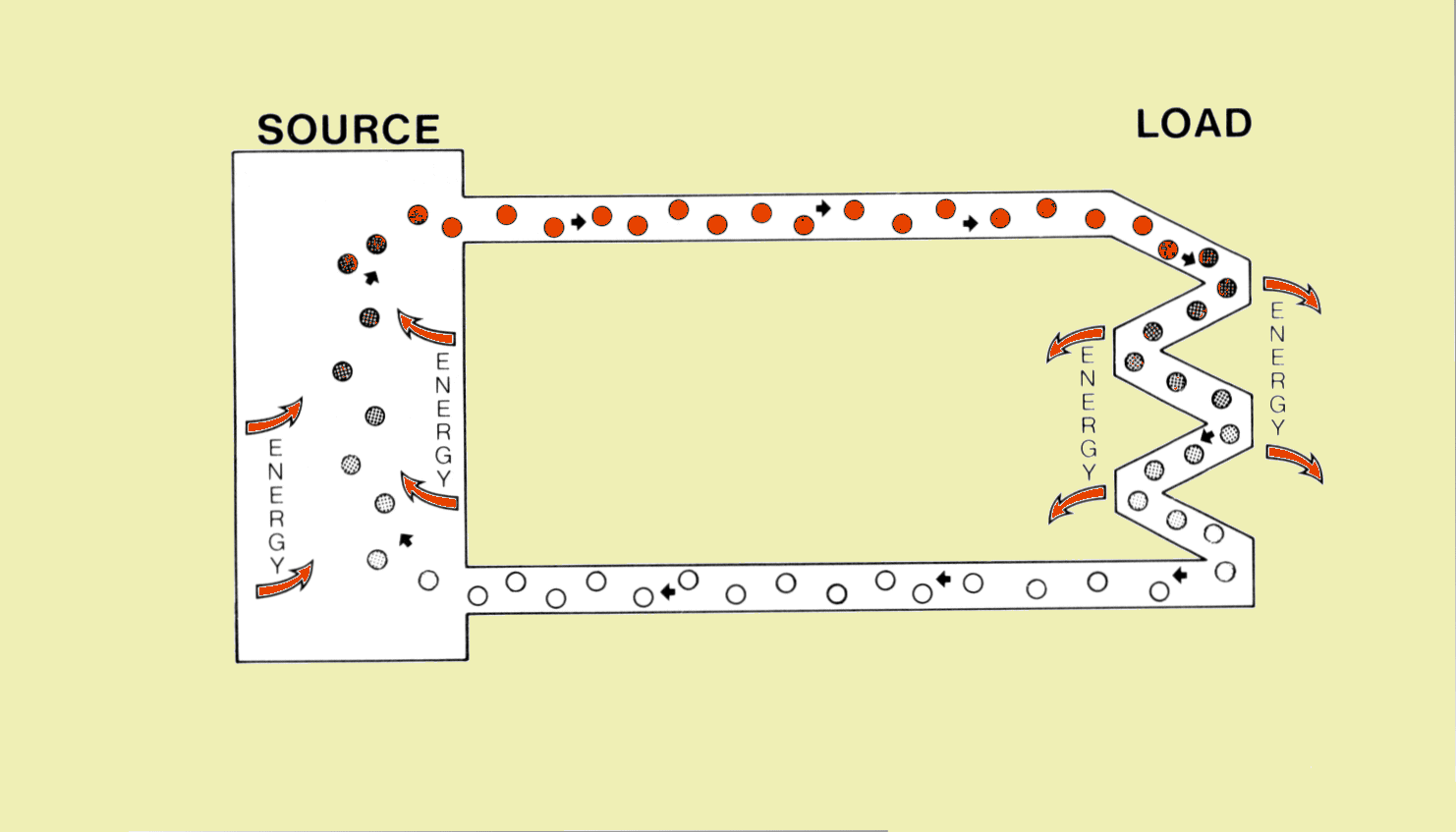A Basic Electrical Circuit

The energy source and load are connected with two conductors over which the electrons can freely move.  The ideal conductor offers no resistance to the flow of the electrons.  If the conductor offers significant resistance, the electrons lose some of their energy there.  The lost energy is converted into heat.  Electrical circuits neither create nor destroy electrons.  The electrons are always present within the conductive materials.  Energy is given to and taken from the electrons as they move around the circuit.

The energy carried by the electrons is a form of potential energy.  Even though the electrons are moving through the conductors, their velocity is not sufficient to give them significant kinetic energy.  When electrons are moving through free space, they can carry significant kinetic energy, but they cannot when they are moving through solid conductors.  In the typical electrical circuit, one conductor has higher potential energy than the other conductor.  In principle, the energy source elevates the electrons to the higher potential energy level which they maintain until they give up the energy in passing through the load device.  The electrons at the lower potential level return to the energy source to repeat the process.

The connection points (terminals) between the source and load devices and the conductors are designated as either positive or negative.  The electrons exit the source at the negative terminal and enter the negative terminal of the load. They then exit the positive terminal of the load device and enter the source at the positive terminal.  In principle, the negative conductor contains the electrons at the high potential energy level.  The positive conductor contains the electrons that have lost their energy and are returning to the source.  In direct current (DC) circuits the polarities do not change. However, in alternating current (AC) circuits the polarity of the conductors is constantly alternating between negative and positive.

# ELECTRICAL QUANTITIES

Each electron passing through the circuit carries a very small amount of energy.  However, by collective effort, electrons can transport a tremendous amount of energy.  The amount of energy transferred by an electrical circuit depends on the quantity of electrons and the energy carried by each.  We now consider these specific electrical quantities and their associated units.

# Current

When an electrical circuit is in operation, electrons are continuously moving or flowing through the conductor.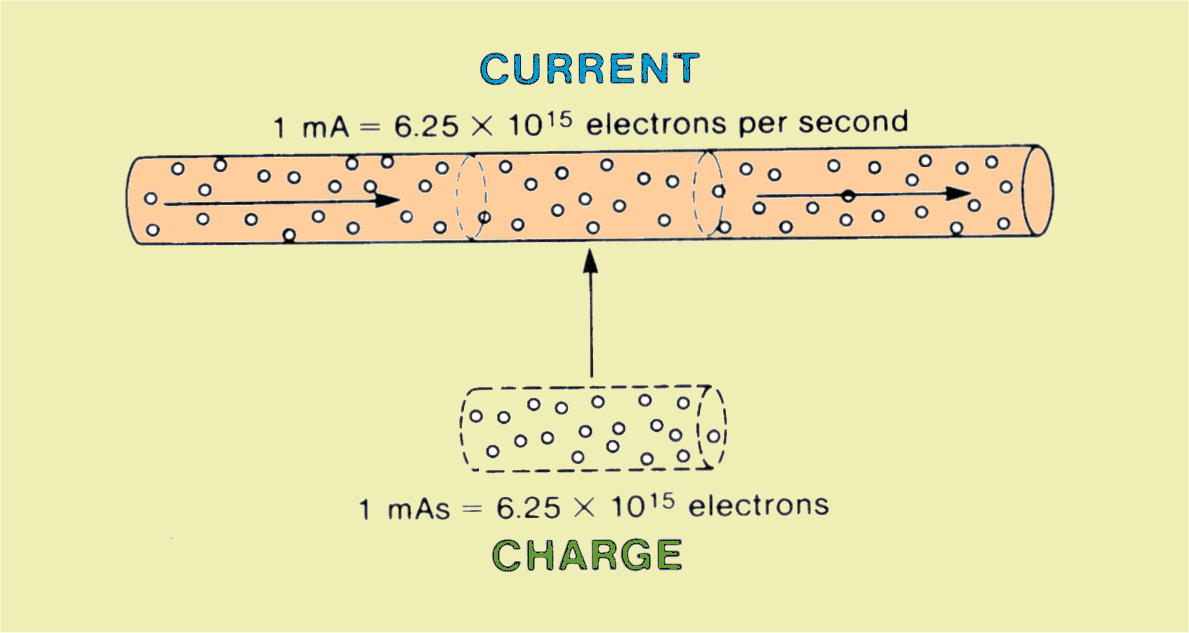Electrical Current and Charge

The number of electrons that move past a given point per second is referred to as the current.  Since, in the typical circuit, the number of electrons per second is quite large, a more useful unit than this number is desirable.  The basic unit of current is the ampere (A).  One ampere is defined as the flow of 6.25 x 1018 electrons per second.  In x-ray machines, the current is typically a fraction of 1 A, and the milliampere (mA) is a more appropriate unit.  As indicated here, a current of 1 mA is equal to the flow of 6.25 x 1015 electrons per second past a given point.  The current that flows through an x-ray tube is generally referred to as the "MA." When used to mean the quantity, it is written as MA. When used as the unit, milliampere, it is written as mA.

In addition to the rate at which electrons are flowing through a circuit, i.e., the current, it is often necessary to know the total quantity in a given period of time.  In x-ray work the most appropriate unit for specifying electron quantity is the milliampere-second (mAs).  The total quantity of electrons passing a point (MAS) is the product of the current (MA) and the time in seconds (S).  Since a current of l mA is a flow of electrons per second, it follows that 1 mAs is a cluster of 6.25 x 1015 electrons, as shown above .

It should be recalled that all electrons carry a negative electrical charge of the same size.  In some situations the quantity of electrons might be specified in terms of the total electrical charge.  If extra electrons are added to an object, it is said to have acquired a negative charge.  However, if some of the free electrons are removed from an object, a positive charge is created.  In either case, the total charge on the object is directly proportional to the number of electrons moved. Generally speaking, charge is a way of describing a quantity of electrons.  The basic unit of charge is the coulomb (C), which is equivalent to the total charge of 6.26 x 1018 electrons; 1 C is equivalent to 1,000 mAs.

We pointed out earlier that electrons could exist at different potential energy levels, because of either their different positions within the atom or their different locations within an electrical circuit.  Consider the two wires or conductors shown here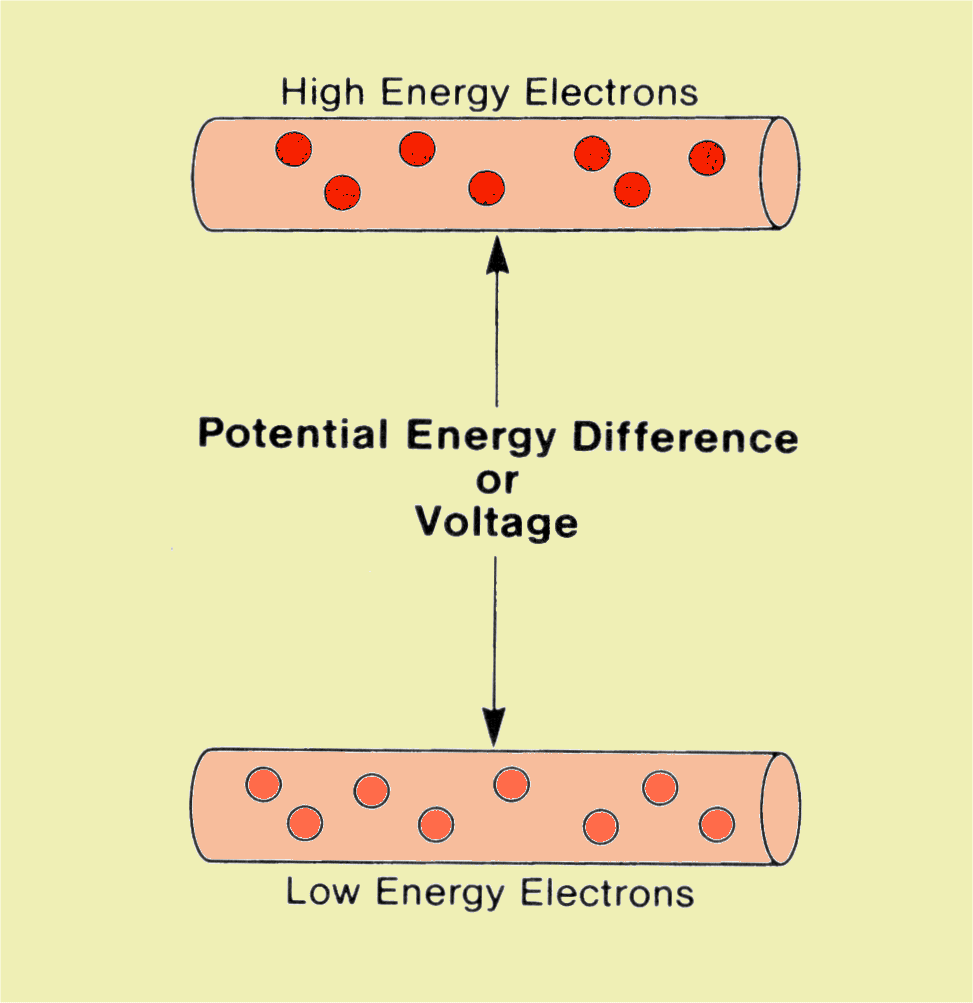Electron Potential Energy or Voltage

The electrons contained in one of the conductors are at a higher potential energy level than the electrons in the other.  Generally, the electrons in the negative conductor are considered to be at the higher energy level.  An electrical quantity that indicates the difference in electron potential energy between two points within a circuit is the voltage, or potential difference, suggesting a difference in potential energy.  The unit used for voltage, or potential difference, is the volt.  The difference in electron potential energy between two conductors is directly proportional to the voltage.  Each electron will have an energy difference of 1 eV for each volt.  It is the quantity of energy that an electron gains or loses, depending on direction, when it moves between two points in a circuit that are 1 V apart.  In the basic x-ray machine circuit the voltage is in the order of thousands of volts (kilovolts) and is often referred to as the KV.  When used to mean the quantity, voltage or potential, it is written KV or KVp. When used as the unit, it is written as kV or kVp.

Electrical Power

Power is the quantity that describes the rate at which energy is transferred.  The watt is the unit of power and is equivalent to an energy transfer rate of 1 J/second.  The power in an electrical circuit is proportional to the energy carried by each electron (voltage) and the rate of electron flow (current). The specific relationship is

Power (watts) = Voltage (volts) x Current (amperes).

# Total Energy

The amount of energy that an electrical circuit transfers depends on the voltage, current, and the duration (time) of the energy transfer. The fundamental unit of energy is the joule. The relationship of total transferred energy to the other electrical quantities is

Energy (joules) = Voltage (volts) x Current (amperes) x Time (seconds).

The basic circuit shown here is found in all x-ray machines.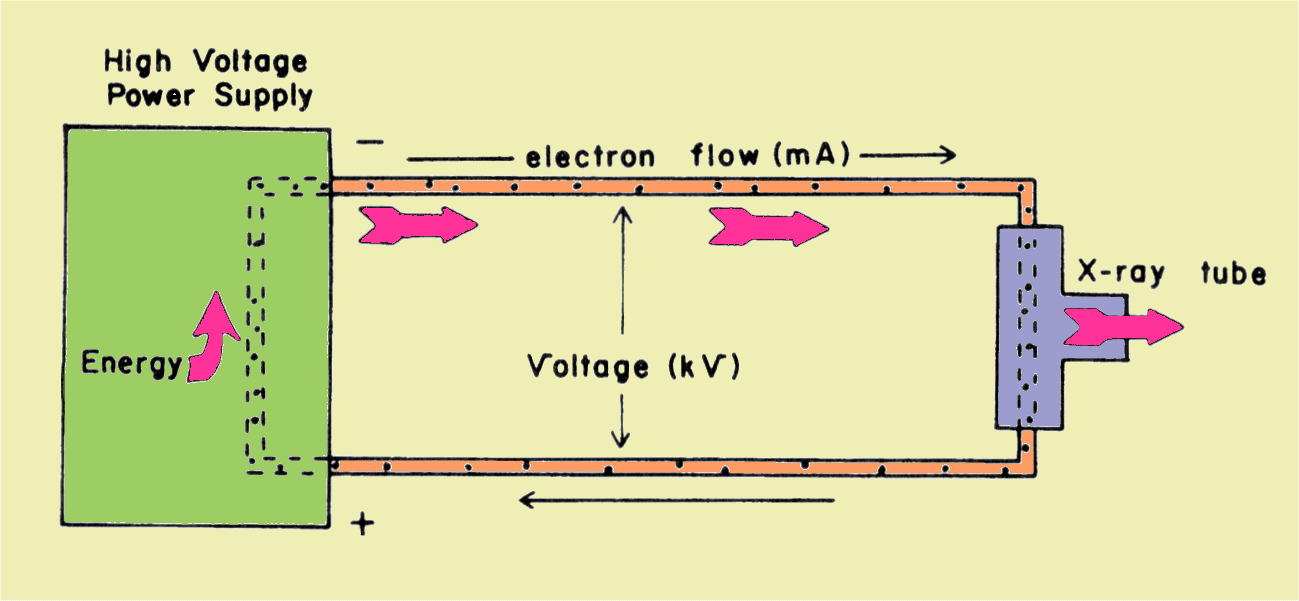The X-Ray Circuit

The power supply that gives energy to the electrons and pumps them through the circuit is discussed in a later Chapter. The voltage between the two conductors in the x-ray circuit is typically in the range of 30,000 V to 120,000 V (30 kV to 120 kV), and in radiology this kilovoltage is generally adjustable and an appropriate value can be selected by the operator of the x-ray equipment.

In this circuit, the x-ray tube is the load.  It is the place where the electrons lose their energy.  The energy lost by electrons in passing through an x-ray tube is converted into heat and x-ray energy.

In some electrical circuits, the voltage and current remain constant with respect to time, and the current always flows in the same direction.  These are generally designated as direct current (DC) circuits.  A battery is an example of a power supply that produces a direct current.

Some power supplies, however, produce voltages that constantly change with time.  Since, in most circuits, the current is more or less proportional to the voltage, it also changes value.  In most circuits of this type, the voltage periodically changes polarity and the current changes or alternates direction of flow.  This is an alternating current (AC) circuit.  The electricity distributed by power companies is AC.  There are certain advantages to AC in that transformers can be used for stepping voltages up or down, and many motors are designed for AC operation.

If a graph of the instantaneous values of either the AC voltage or current is plotted with respect to time, it will generally be similar to the one shown here.  This representation of the voltage with respect to time is known as the waveform.  Most AC power sources produce voltages with the sine-wave waveform as shown here.  This name is derived from the mathematical description of its shape.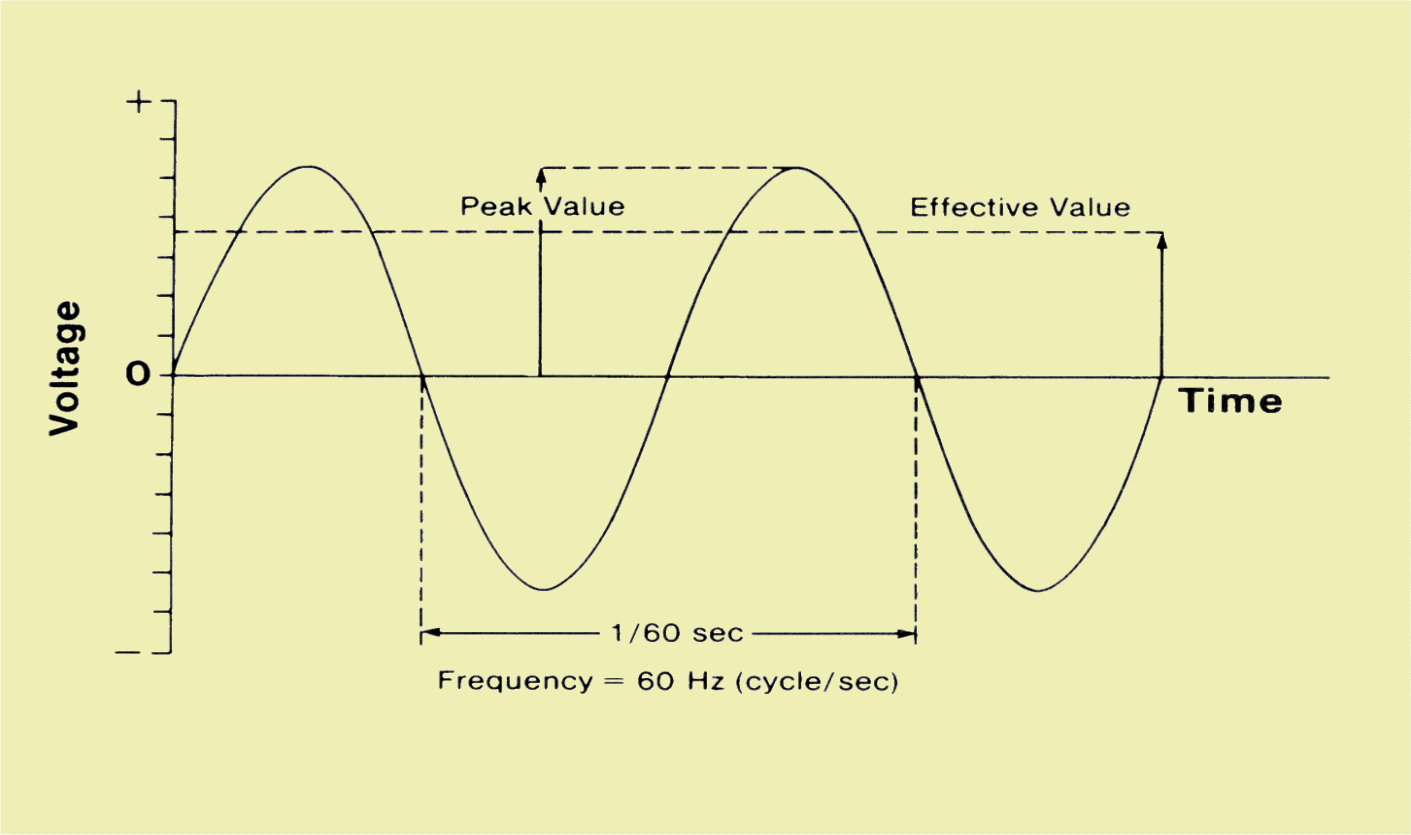Waveform of an Alternating Voltage

One characteristic of an alternating voltage is its frequency.  The frequency is the rate at which the voltage changes through one complete cycle.  The time of one complete cycle is the period; the frequency is the reciprocal of the period.  For example, the electricity distributed in the United States goes through one complete cycle in 0.0166 seconds and has a frequency of 60 cycles per second.  The unit for frequency is the hertz, which is 1 cycle per second.

During one voltage cycle, the voltage changes continuously.  At two times during the period it reaches a peak, but remains there for a very short time.  This means that for most of the period the circuit voltage is less than the peak value.  For the purpose of energy and power calculations, an effective voltage value, rather than the peak value, should be used.  For the sine-wave voltage, the effective value is 70.7% (0.707) of the peak voltage.  This is the waveform factor, and its value depends on the shape of the voltage waveform.